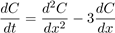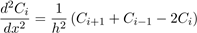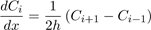# We Helped With This Differential Equations Homework: Have A Similar One?

SOLVEDCategory Math Differential Equations Undergraduate Solved Differential Equations Help

## Short Assignment Requirements

Modelling a convection-diffusion formula which is dependent on both time and space with Euler-Forward, using initial conditions and boundary conditions

## Assignment Description

Exercise

We consider a model with diffusion and convection for some concentration C:.

with initial condition C(t = 0,x) = 1 for 2 ≤ x ≤ 3 and C(t = 0,x) = 0 otherwise. We use boundary conditions C(t,x = 0) = C(t,x = 6) = 0, but C(t,x = 0) = 1 for 4 ≤ t ≤ 5. This is a partial differential equation where C depends on both time t and space x. It cannot be solved easily analytically. Let us make a numerical solution with forward-Euler. For this, we are going to discretize this system as follows. We compute the solution C at discrete points xi in space only. These points lie at a distance h, so xi = ih with i = 0,1,2,...,N with N = 6/h. With this notation we can write Ci = C(t,xi). Then we approximate the spatial derivatives with the following formulas,Write down the differential equations for C1,C2,C3,C4,C5 if h = 1. Do this in a matrix form C0 = AC + b so that C = (C1....CN−1). That is, give the matrix A and vector b. Also specify the initial condition C(t = 0).

Use dt = 0.25 to simulate the system for 0 ≤ t ≤ 10. Make a contourplot of your solution with t and x on the axes. Also plot the solution for t = 1,5,10.

Now use h = 0.25 and simulate the system for 0 ≤ t ≤ 10. You cannot use the same dt, but need a smaller value. Experiment with smaller values until your simulation makes sense. Hint: you may for-loops to initialize the matrix A once. Make the same plots as before.

1

Is it free to get my assignment evaluated?

Yes. No hidden fees. You pay for the solution only, and all the explanations about how to run it are included in the price. It takes up to 24 hours to get a quote from an expert. In some cases, we can help you faster if an expert is available, but you should always order in advance to avoid the risks. You can place a new order here.

How much does it cost?

The cost depends on many factors: how far away the deadline is, how hard/big the task is, if it is code only or a report, etc. We try to give rough estimates here, but it is just for orientation (in USD):

 Regular homework \$20 - \$150 Advanced homework \$100 - \$300 Group project or a report \$200 - \$500 Mid-term or final project \$200 - \$800 Live exam help \$100 - \$300 Full thesis \$1000 - \$3000

How do I pay?

Credit card or PayPal. You don't need to create/have a Payal account in order to pay by a credit card. Paypal offers you "buyer's protection" in case of any issues.

Why do I need to pay in advance?

We have no way to request money after we send you the solution. PayPal works as a middleman, which protects you in case of any disputes, so you should feel safe paying using PayPal.

Do you do essays?

No, unless it is a data analysis essay or report. This is because essays are very personal and it is easy to see when they are written by another person. This is not the case with math and programming.

Why there are no discounts?

It is because we don't want to lie - in such services no discount can be set in advance because we set the price knowing that there is a discount. For example, if we wanted to ask for \$100, we could tell that the price is \$200 and because you are special, we can do a 50% discount. It is the way all scam websites operate. We set honest prices instead, so there is no need for fake discounts.

Do you do live tutoring?

No, it is simply not how we operate. How often do you meet a great programmer who is also a great speaker? Rarely. It is why we encourage our experts to write down explanations instead of having a live call. It is often enough to get you started - analyzing and running the solutions is a big part of learning.

What happens if I am not satisfied with the solution?

Another expert will review the task, and if your claim is reasonable - we refund the payment and often block the freelancer from our platform. Because we are so harsh with our experts - the ones working with us are very trustworthy to deliver high-quality assignment solutions on time.

Customer Feedback

"Thanks for explanations after the assignment was already completed... Emily is such a nice tutor! "

Order #13073